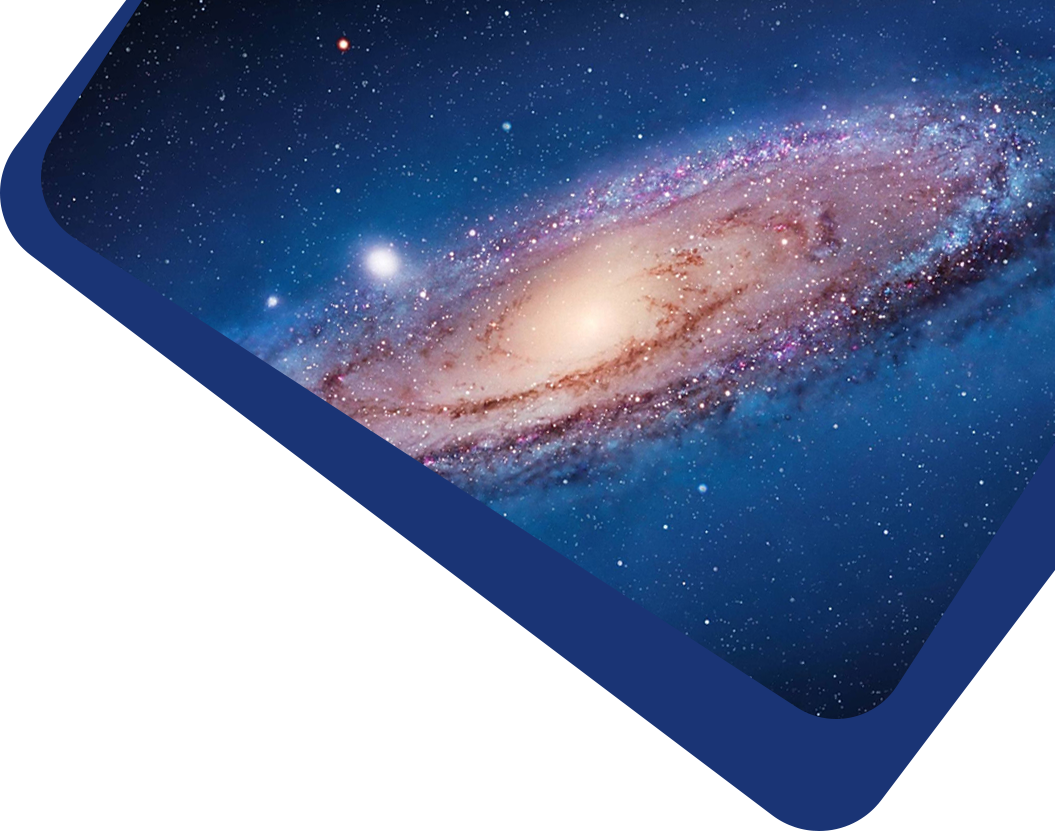Vol 23, No 1

## Photometric Properties and Stellar Parameters of the Rapidly Rotating Magnetic Early-B Star HD 345439

#### Dong-Xiang Shen, Jin-Zhong Liu, Chun-Hua Zhu, Guo-Liang Lü, Yu Zhang, Cheng-Long Lü, Hao-Zhi Wang, Lei Li, Xi-Zhen Lu, Jin-Long Yu et al

Abstract

We first present the multicolor photometry results of the rapidly rotating magnetic star HD 345439 using the Nanshan One-meter Wide-field Telescope. From the photometric observations, we derive a rotational period of 0.7699 ± 0.0014 day. The light curves of HD 345439 are dominated by the double asymmetric S-wave feature that arises from the magnetic clouds. Pulsating behaviors are not observed in Sector 41 of the Transiting Exoplanet Survey Satellite. No evidence is found of the occurrence of centrifugal breakout events neither in the residual flux nor in the systematic variations at the extremum of the light curve. Based on the hypothesis of the Rigidly Rotating Magnetosphere model, we restrict the magnetic obliquity angle β and the rotational inclination angle i so that they satisfy the approximate relation β + i ≈ 105°. The color excess, extinction, and luminosity are determined to be E(B−V ) = 0.745 ± 0.016 mag, AV = 2.31 ± 0.05 mag, and $$\log(L/L_{\odot})= 3.82\pm 0.1$$ dex, respectively. Furthermore, we derive the effective temperature as Teff = 22 ± 1 kK and the surface gravity as log g = 4.00 ± 0.22. The mass $$M= 7.24_{-1.24}^{+1.75} M_{\odot}$$, radius $$R=4.44_{-1.93}^{+2.68} R_{\odot}$$, and age $$\tau_{\rm age}= 23.62_{-21.97}^{+4.24}$$ Myr are estimated from the Hertzsprung–Russell diagram.

Keywords

stars: chemically peculiar – stars: early-type – stars: fundamental parameters – stars: magnetic field

Full Text
Refbacks